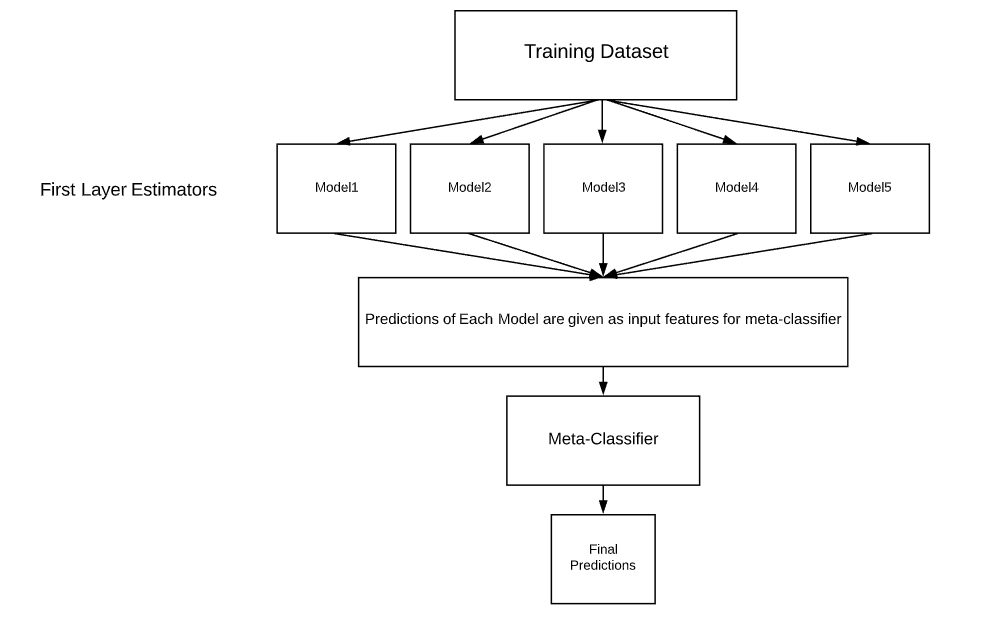1595338862

# Understanding Model Pruning in TensorFlow

In this article, we’ll work through an example as we apply pruning and view the effect on the final model size and prediction errors.

## Import the Usual Suspects

Our first step is to get a couple of imports out of the way:

• `Os` and `Zipfile` will help us in assessing the size of the models.
• `tensorflow_model_optimization` for model pruning.
• `load_model` for loading a saved model.
• and of course `tensorflow` and `keras`.

Finally, we initialize TensorBoard so that we’ll able to visualize the models:

``````import os
import zipfile
import tensorflow as tf
import tensorflow_model_optimization as tfmot
from tensorflow import keras
``````

## Dataset Generation

For this experiment, we’ll generate a regression dataset using scikit-learn. Thereafter, we split the dataset into a training and test set:

``````from sklearn.datasets import make_friedman1
X, y = make_friedman1(n_samples=10000, n_features=10, random_state=0)

from sklearn.model_selection import train_test_split
X_train, X_test, y_train, y_test = train_test_split(X, y, test_size=0.33, random_state=42)
``````

## Model Without Pruning

We’ll create a simple neural network to predict the target variable `y`. We’ll then check the mean squared error. After this, we’ll compare this with the entire model pruned, and then with just the `Dense` layer pruned.

#heartbeat #neural-networks #model-optimization #tensorflow #machine-learning

## Buddha Community1595338862

## Understanding Model Pruning in TensorFlow

In this article, we’ll work through an example as we apply pruning and view the effect on the final model size and prediction errors.

## Import the Usual Suspects

Our first step is to get a couple of imports out of the way:

• `Os` and `Zipfile` will help us in assessing the size of the models.
• `tensorflow_model_optimization` for model pruning.
• `load_model` for loading a saved model.
• and of course `tensorflow` and `keras`.

Finally, we initialize TensorBoard so that we’ll able to visualize the models:

``````import os
import zipfile
import tensorflow as tf
import tensorflow_model_optimization as tfmot
from tensorflow import keras
``````

## Dataset Generation

For this experiment, we’ll generate a regression dataset using scikit-learn. Thereafter, we split the dataset into a training and test set:

``````from sklearn.datasets import make_friedman1
X, y = make_friedman1(n_samples=10000, n_features=10, random_state=0)

from sklearn.model_selection import train_test_split
X_train, X_test, y_train, y_test = train_test_split(X, y, test_size=0.33, random_state=42)
``````

## Model Without Pruning

We’ll create a simple neural network to predict the target variable `y`. We’ll then check the mean squared error. After this, we’ll compare this with the entire model pruned, and then with just the `Dense` layer pruned.

#heartbeat #neural-networks #model-optimization #tensorflow #machine-learning1621931885

## How TensorFlow Lite Fits In The TinyML Ecosystem

TensorFlow Lite has emerged as a popular platform for running machine learning models on the edge. A microcontroller is a tiny low-cost device to perform the specific tasks of embedded systems.

In a workshop held as part of Google I/O, TensorFlow founding member Pete Warden delved deep into the potential use cases of TensorFlow Lite for microcontrollers.

Further, quoting the definition of TinyML from a blog, he said:

“Tiny machine learning is capable of performing on-device sensor data analytics at extremely low power, typically in the mW range and below, and hence enabling a variety of ways-on-use-case and targeting battery operated devices.”

#opinions #how to design tinyml #learn tinyml #machine learning models low cost #machine learning models low power #microcontrollers #tensoflow latest #tensorflow lite microcontrollers #tensorflow tinyml #tinyml applications #tinyml models1623906928

## What is Model Stacking?

Model Stacking is a way to improve model predictions by combining the outputs of multiple models and running them through another machine learning model called a meta-learner. It is a popular strategy used to win kaggle competitions, but despite their usefulness they’re rarely talked about in data science articles — which I hope to change.

Essentially a stacked model works by running the output of multiple models through a “meta-learner” (usually a linear regressor/classifier, but can be other models like decision trees). The meta-learner attempts to minimize the weakness and maximize the strengths of every individual model. The result is usually a very robust model that generalizes well on unseen data.

The architecture for a stacked model can be illustrated by the image below:#tensorflow #neural-networks #model-stacking #how to use “model stacking” to improve machine learning predictions #model stacking #machine learning1623228736

## 5 Steps to Passing the TensorFlow Developer Certificate

Deep Learning is one of the most in demand skills on the market and TensorFlow is the most popular DL Framework. One of the best ways in my opinion to show that you are comfortable with DL fundaments is taking this TensorFlow Developer Certificate. I completed mine last week and now I am giving tips to those who want to validate your DL skills and I hope you love Memes!

1. Do the DeepLearning.AI TensorFlow Developer Professional Certificate Course on Coursera Laurence Moroney and by Andrew Ng.

2. Do the course questions in parallel in PyCharm.

#tensorflow #steps to passing the tensorflow developer certificate #tensorflow developer certificate #certificate #5 steps to passing the tensorflow developer certificate #passing1623906637

## Beginners Guide to Debugging TensorFlow Models

If you are new to working with a deep learning framework, such as TensorFlow, there are a variety of typical errors beginners face when building and training models. Here, we explore and solve some of the most common errors to help you develop a better intuition for debugging in TensorFlow.

TensorFlow is one of the most famous deep learning models, and it is easy to learn. This article will discuss the most common errors a beginner can face while learning TensorFlow, the reasons, and how to solve these errors. We will discuss the solutions and also what experts from StackOverflow say about them.

#### Example ## 4: Unknown Loss function

#2021 jun tutorials #overviews #beginners #deep learning #tensorflow #beginners guide to debugging tensorflow models